• np.around()函数用于返回四舍五入后的值，可指定精度。 np.floor()函数用于以元素方式返回输入的下限。 np.ceil()函数用于以元素方式返回输入的上限。 1、np.around np.around(a, decimals=0, out=None ) 对于...
欢迎关注WX公众号：【程序员管小亮】
np.around()函数用于返回五舍六入后的值，可指定精度。
np.floor()函数用于以元素方式返回输入的下限。
np.ceil()函数用于以元素方式返回输入的上限。
np.where()函数根据 condition 从 x 和 y 中选择元素，当为 True 时，选 x，否则选 y。
1、np.around
np.around(a,
decimals=0,
out=None
)

对于正好在舍入小数值之间的值，NumPy舍入到最接近的偶数值。当超过5时候(不包含5)，才会进位！因此，1.5和2.5轮到2.0，-0.5和0.5轮到0.0等。由于IEEE浮点标准中的小数部分的不精确表示以及当以10的幂进行缩放时引入的误差，结果也可能是令人惊讶的。
参数：

a ： array_like。输入数据。

decimals ： int，可选。要舍入的小数位数（默认值：0）。如果小数为负数，则指定小数点左侧的位置数。

out ： ndarray，可选。替代输出数组，用于放置结果。它必须具有与预期输出相同的形状，但如果需要，将输出输出值的类型。

返回：

rounded_array ： ndarray。与a相同类型的数组，包含舍入值。除非指定了out，否则将创建一个新数组。返回对结果的引用。复数的实部和虚部分别舍入。舍入浮点数的结果是浮点数。

例子1：
>>> np.around([0.37, 1.64])
array([ 0.,  2.])
>>> np.around([0.37, 1.64], decimals=1)
array([ 0.4,  1.6])
>>> np.around([.5, 1.5, 2.5, 3.5, 4.5]) # rounds to nearest even value
array([ 0.,  2.,  2.,  4.,  4.])
>>> np.around([1,2,3,11], decimals=1) # ndarray of ints is returned
array([ 1,  2,  3, 11])
>>> np.around([1,2,3,11], decimals=-1)
array([ 0,  0,  0, 10])

2、np.floor
np.floor(x, /, out=None, *, where=True,
casting='same_kind', order='K', dtype=None,
subok=True[, signature, extobj]) = <ufunc 'floor'>

参数：

x ： array_like。输入数据。

out ： ndarray，None或ndarray和None的元组，可选。存储结果的位置。如果提供，它必须具有输入广播的形状。如果未提供或None，则返回新分配的数组。元组（仅可作为关键字参数）的长度必须等于输出的数量。

where ： array_like，可选。值True表示计算该位置的ufunc，值False表示仅将值保留在输出中。

返回：

y ： ndarray或标量。x中每个元素的底限。如果x是标量，则这是标量。

例子2：
>>> a = np.array([-2.5, -1.7, -1.5, -0.2, 0.2, 1.5, 1.7, 2.0])
>>> np.floor(a)
array([-3., -2., -2., -1.,  0.,  1.,  1.,  2.])

3、np.ceil
np.ceil(x, /, out=None, *, where=True,
casting='same_kind', order='K', dtype=None,
subok=True[, signature, extobj]) = <ufunc 'ceil'>

参数：

x ： array_like。输入数据。

out ： ndarray，None或ndarray和None的元组，可选。存储结果的位置。如果提供，它必须具有输入广播的形状。如果未提供或None，则返回新分配的数组。元组（仅可作为关键字参数）的长度必须等于输出的数量。

where ： array_like，可选。值True表示计算该位置的ufunc，值False表示仅将值保留在输出中。

返回：

y ： ndarray或标量。x中每个元素的上限，带floatdtype。如果x是标量，则这是标量。

例子3：
>>> a = np.array([-1.7, -1.5, -0.2, 0.2, 1.5, 1.7, 2.0])
>>> np.ceil(a)
array([-1., -1., -0.,  1.,  2.,  2.,  2.])

4、np.where
np.where(condition,
x,
y
)

如果只给出条件，则返回condition.nonzero()。
参数：

condition：array_like，bool。如果为True，则产生x，否则产生y。

x，y：array_like，可选。要从中选择的值。x，y和条件需要可以广播broadcastable到某种形状。

返回：

out：ndarray或ndarray元组。如果同时指定了x和y，则输出数组包含x的元素，其中condition为True，其他元素来自 y。如果只给出条件，则返回元组condition.nonzero()，条件为True 的索引。

例子4：
# 如果给出x和y并且输入数组是1-D，where则相当于：

[xv if c else yv for (c,xv,yv) in zip(condition,x,y)]

例子5：
>>> np.where([[True, False], [True, True]],
...          [[1, 2], [3, 4]],
...          [[9, 8], [7, 6]])
array([[1, 8],
[3, 4]])
>>> np.where([[0, 1], [1, 0]])
(array([0, 1], dtype=int64), array([1, 0], dtype=int64))

例子6：
>>> x = np.arange(9.).reshape(3, 3)
>>> print(x)
[[0. 1. 2.]
[3. 4. 5.]
[6. 7. 8.]]

>>> np.where( x > 5 )
(array([2, 2, 2]), array([0, 1, 2]))
>>> x[np.where( x > 3.0 )]               # Note: result is 1D.
array([ 4.,  5.,  6.,  7.,  8.])
>>> np.where(x < 5, x, -1)               # Note: broadcasting.
array([[ 0.,  1.,  2.],
[ 3.,  4., -1.],
[-1., -1., -1.]])

>>> goodvalues = [3, 4, 7]
>>> ix = np.isin(x, goodvalues)
>>> ix
array([[False, False, False],
[ True,  True, False],
[False,  True, False]])
>>> np.where(ix)
(array([1, 1, 2]), array([0, 1, 1]))

python课程推荐。展开全文numpy
• numpy.where() 有两种用法： 1. np.where(condition, x, y) 满足条件(condition)，输出x，不满足输出y。 import numpy as np a = np.arange(10) print(a) aa = np.where(a, 1, -1) print(aa) aaa = np.where(a > ...
numpy.where() 有两种用法：
1. np.where(condition, x, y)
满足条件(condition)，输出x，不满足输出y。
import numpy as np
a = np.arange(10)
print(a)
aa = np.where(a, 1, -1)
print(aa)
aaa = np.where(a > 5, 1, -1)
print(aaa)

[0 1 2 3 4 5 6 7 8 9]
[-1  1  1  1  1  1  1  1  1  1]
[-1 -1 -1 -1 -1 -1  1  1  1  1]

np.where([[True,False], [True,True]],    # 官网上的例子
[[1,2], [3,4]],
[[9,8], [7,6]])

array([[1, 8],
[3, 4]])


上面这个例子的条件为[[True,False], [True,False]]，分别对应最后输出结果的四个值。第一个值从[1,9]中选，因为条件为True，所以是选1。第二个值从[2,8]中选，因为条件为False，所以选8，后面的以此类推。

同理，再看下面的一个例子：

python
a = 10
np.where([[a > 5,a < 5], [a == 10,a == 7]],
[["chosen","not chosen"], ["chosen","not chosen"]],
[["not chosen","chosen"], ["not chosen","chosen"]])

array([['chosen', 'chosen'],
['chosen', 'chosen']], dtype='<U10')

2. np.where(condition)
只有条件 (condition)，没有x和y，则输出满足条件 (即非0) 元素的坐标 。这里的坐标以tuple的形式给出，通常原数组有多少维，输出的tuple中就包含几个数组，分别对应符合条件元素的各维坐标。
一维的情况:
a = np.array([2, 4, 6, 8, 10])
print(np.where(a > 5))
print(type(np.where(a > 5)))
print(a[np.where(a > 5)])
print(type(a[np.where(a > 5)]))

(array([2, 3, 4], dtype=int64),)
<class 'tuple'>
[ 6  8 10]
<class 'numpy.ndarray'>

二维的情况：
a = np.arange(27).reshape(3,3,3)
a

array([[[ 0,  1,  2],
[ 3,  4,  5],
[ 6,  7,  8]],

[[ 9, 10, 11],
[12, 13, 14],
[15, 16, 17]],

[[18, 19, 20],
[21, 22, 23],
[24, 25, 26]]])

np.where(a > 5)

(array([0, 0, 0, 1, 1, 1, 1, 1, 1, 1, 1, 1, 2, 2, 2, 2, 2, 2, 2, 2, 2],
dtype=int64),
array([2, 2, 2, 0, 0, 0, 1, 1, 1, 2, 2, 2, 0, 0, 0, 1, 1, 1, 2, 2, 2],
dtype=int64),
array([0, 1, 2, 0, 1, 2, 0, 1, 2, 0, 1, 2, 0, 1, 2, 0, 1, 2, 0, 1, 2],
dtype=int64))

a[np.where(a > 5)]
array([ 6,  7,  8,  9, 10, 11, 12, 13, 14, 15, 16, 17, 18, 19, 20, 21, 22,
23, 24, 25, 26])

所以满足条件的坐标为(0, 2, 0), (0, 2, 1)…
所以np.where会输出每个元素的对应的坐标，因为原数组有三维，所以tuple中有三个数组。


展开全文• np.where(条件)，若条件满足，返回索引值 np.where(条件，x，y)，若条件满足，返回x，否则返回y
np.where(条件)，若条件满足，返回索引值
np.where(条件，x，y)，若条件满足，返回x，否则返回y



展开全文numpy
• np.where()函数使用 np.where 函数是三元表达式 x if condition else y的矢量化版本 result = np.where(cond,x,y) 当符合条件时是x，不符合是y，常用于根据一个数组产生另一个新的数组。 栗子：假设有一个...
tqdm模块：用来显示工作的进度条
from tqdm import tqdm
import time
bar = tqdm(['p1','p2','p3','p4','p5'])
for b in bar:
time.sleep(0.5)
bar.set_description("处理{0}中".format(b))

进度条一直往下滚动的问题，可以通过加ncols参数解决，一般设置ncols = 80即可
bar.update(1)  #每次更新进度条的长度
pandas pd.concat()函数
pd.concat(objs, axis=0, join='outer', join_axes=None, ignore_index=False,
keys=None, levels=None, names=None, verify_integrity=False)

参数说明
objs: series，dataframe或者是panel构成的序列lsit
axis： 需要合并链接的轴，0是行，1是列
join：连接的方式 inner，或者outer
1.1 相同字段的表首尾相接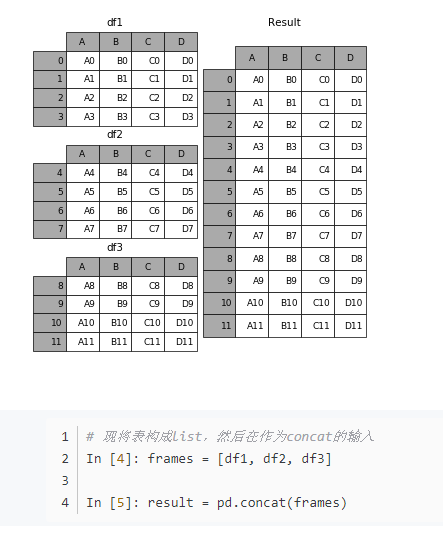pandas修改列名：
new_name_list = ['A', 'B', 'C', 'D']
df.columes = new_name_list

DataFrame的基础属性
df.shape ——行数 列数
df.dtypes——列数据类型
df.ndim ——数据维度
df.index——行索引
df.columns——列索引
df.values——对象值，二维ndarray数组
DataFrame整体情况
df.head(10)——显示前10行，默认是5行
df.tail()——显示末尾几行，默认是5
df.info()——相关系数，如行数，列数，列索引、列非空值个数，列类型，内存占用
df.describe()——快速统计结果，计数、均值、标准差、最大值、四分数、最小值
pandas格式化输出时间
transactions['date_formatted']=pd.to_datetime(transactions['date'], format='%d.%m.%Y')

%Y	4位数的年
%y	2位数的年
%m	2位数的月[01,12]
%d	2位数的日[01，31]
%H	时（24小时制）[00,23]
%l	时（12小时制）[01,12]
%M	2位数的分[00,59]
%S	秒[00,61]有闰秒的存在
%w	用整数表示的星期几[0（星期天），6]
%F	%Y-%m-%d简写形式例如，2017-06-27
%D	%m/%d/%y简写形式
pd.merge()函数
使用过sql语言的话，一定对join，left join， right join等非常熟悉，在pandas中，merge的作用也非常类似。
如：pd.merge(df1, df2) 找到一个外键，然后将两条数据合并成一条。
left: 按照left的dataframe为基准，右边值为空的话就默认nan
train = pd.merge(train, t, on='ship', how='left')

Pandas里Groupby的apply用法df.groupby('key').apply(lamdba x: x['v'].sum())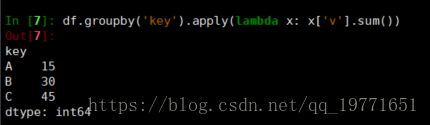下面是apply的另外一种用途，即保证原数据的前提下把分组后求得的和与原数据合并，首先我们先定义一个函数

def func_sum(df):
df['v_sum'] = df['v'].sum()
return df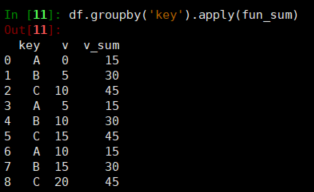可以看到原数据添加了新的一列，这一列的值是每种key对应的v的和，上述自定义函数这类方法更具有灵活性，可以同时求和，平均，最大值，最小值，排序等等.
以上便是对于Groupby中的apply函数的简单介绍，在Groupby中还有一类函数，那就是agg函数，这个函数只能实现特定的聚合操作，比如mean,sum, apply函数可以说是它的泛化，比如你可以用apply实现组内排序，但是agg函数并不能。
Pandas里Groupby的agg函数用法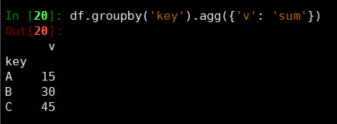agg函数传入一个字典，键指对应的列名，值指聚合函数如{‘sum’, ‘count’, ‘mean’}之类
下面的操作是agg的简化版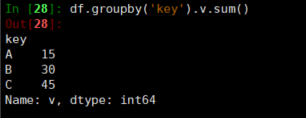np.where()函数使用
np.where 函数是三元表达式 x if condition else y的矢量化版本
result = np.where(cond,x,y)
当符合条件时是x，不符合是y，常用于根据一个数组产生另一个新的数组。
栗子：假设有一个随机数生成的矩阵，希望将所有正值替换为2，负值替换为-2
arr = np.random.randn(4,4)
arr
np.where(arr>0,2,-2)



展开全文• 其中matlab find()函数转换成np.where()函数就遇到了一些麻烦。 一、我们先来看看一维矩阵的对比 1、matlab—— 总结：可以看到在处理一维矩阵时，当find不到要找的值，返回的就是空；当find到了，返回的就是起点为1...
• np.where()函数根据 condition 从 x 和 y 中选择元素，当为 True 时，选 x，否则选 y。 2. 详细介绍 2.1.np.around np.around(a, decimals=0, out=None )   对于正好在舍入小数值之间的值，NumPy舍入到最接近的...python numpy
• 看了很多博客，见到的没有一个给说清楚了，这里做个记录。这是python提供的函数说明：
• Pytorch的torch.where（）函数和numpy中的np.where（）函数使用方法完全是一致的。torch.where（）需要三个参数：torch.where（第一个为判断条件，第二个为满足条件设置的值，第三个为不满足条件设置的值）。等同于...
• np.where()函数 np.where(condition[,x,y]) 参数： 1.三个参数满足条件condition时输出x，不满足时输出y x,y是从中选择的值 2.一个参数np.where(arry)： 输出arry中‘真’值的坐标或者为非零的坐标 例子： import ...
• 近日用到numpy.where()函数，大部分使用方式都能理解，但是在看 >>> x = np.arange(9.).reshape(3, 3) >>> np.where( x > 5 ) (array([2, 2, 2]), array([0, 1, 2])) 的时候就懵逼了，为什么是两个数组？ 后来才...
• ## np.where(cond,x,y)函数

千次阅读 2018-05-23 15:53:22
今天在看特征提取的代码时遇到了np.where()函数，一脸懵逼，查了资料并没有发现什么有价值的内容。知乎上逛了一圈略有收获。记录下来np.where(condiction,x,y)这个函数的三个输入参数分别是条件（可以是矩阵），x,y...
• np.where函数 np.where函数: np.where函数是三元表达式 x if condition else y的矢量化版本。 help(np.where): Help on built-in function wh......